## Monday, January 11, 2010

### Irodov Problem 1.384As seen from the stationary frame of reference (in which N2 is stationary), neutron N1 is on a collision course towards neutron N2 and has a kinetic energy of .The center of inertia of the (N1+N2) system (CG) lies in between N1 and N2 and moves with velocity vCG. As seen from the reference frame of the CG, both neutrons N1 and N2 move towards the CG at equal velocities. This is shown in the Figure above.

The total energy of N1 in the stationary reference frame is given by,We can now compute the velocity v of neutron N1 as,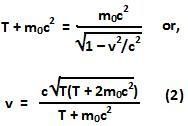Hence, the momentum of neutron N1 and hence the entire system (N1 + N2) and hence, the momentum of the CG as seen from the staionary reference frame is given by,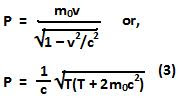The total energy of the entire system (N1 + N2) and hence that of the CG, as seen from the stationary reference frame is given by the sum of kinetic energy of the neutron N1 and the sum of the rest mass energies of each of the neutrons N1 and N2. Thus, the total energy in the system in the stationary reference frame is given by,The invariant quantity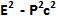can then be computed as,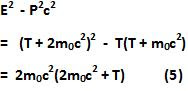This quantity is invariant with respect to the choice of any inertial frame and hence must be the same even in the frame of the center of inertia (CG). The momentum of the CG is 0 in the reference frame of the CG. Hence the energy of the CG, ECG can be computed as,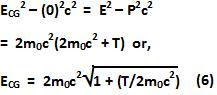The kinetic energy of the system as viewed from a reference frame CG can be obtained by subtracting the rest mass energies of the two protons from total energy as,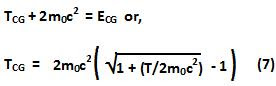As seen from the reference frame of the CG, both neutrons will have the same energy and hence then energy of each neutron in the CG reference frame will be,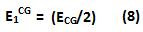We also know from problem 1.383 that for each neutron,Here,is the momentum of the neutron N1 in the reference frame of the CG.

b) The total rest mass of the CG is 2mo. Hence, at velocity VCG, its energy is given by. We can thus, compute the velocity of the CG as,